## Wednesday, April 26, 2017

### Showing multiple conflicting phylogenetic trees in the same plot

Yesterday, an R-sig-phylo subscriber posted the following question:

“For comparison of two trees I can use very nice function `cophyloplot` plotting two trees (left and right) and connecting respective nodes by lines. Very nice and convenient to read. But only for two trees. Displaying multiple trees in multiple comparisons is not very convenient. To display dozens to hundreds of trees there is densitree. Also nice, but for this purpose I don't like its display. I have several trees (~5) and I wish to compare their topologies, show supports (at least for differing nodes) and highlight differences. I thought about some overlay/parallel plotting (similar to the attached image) where there would be complete topologies displayed and incongruences would be easily visible. It would be probably doable by plotting all separate trees by `plot.phylo` and then combining and tuning the figure in some vector editor (like Inkscape). But I hope there is some more automated way to do it.”

The poster also supplied the following image as example: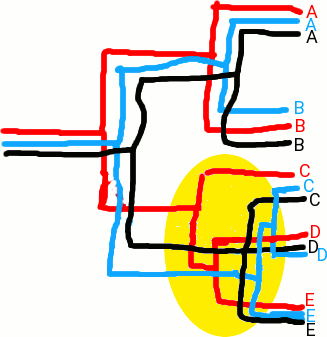Here are a couple of possible solutions using phytools.

Solution 1: Overlay the trees with “inner” node position.

``````library(phytools)
``````
``````## Loading required package: ape
``````
``````## Loading required package: maps
``````
``````trees
``````
``````## 9 phylogenetic trees
``````
``````## get depths of the tree
h<-sapply(trees,function(x) max(nodeHeights(x)))
## test plot the longest tree to get the maximum x dimension
plotTree(trees[[which(h==max(h))]],direction="leftwards")
``````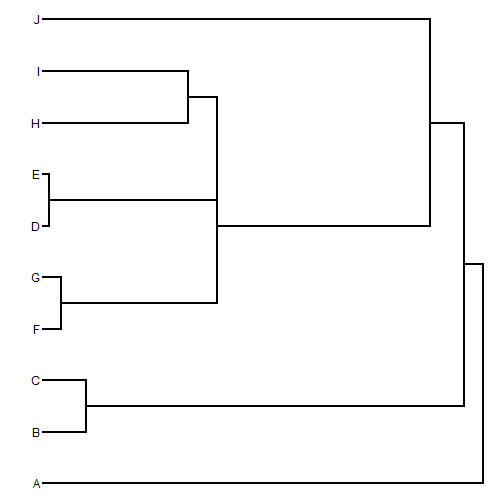``````xlim<-get("last_plot.phylo",envir=.PlotPhyloEnv)\$x.lim
## set tip order based on a majority rule consensus tree
tips<-setNames(1:Ntip(trees[]),
## plot our trees
for(i in 1:length(trees)){
plotTree(trees[[i]],color=make.transparent("blue",0.2),
mar=c(4.1,1.1,1.1,1.1),nodes="inner",
ftype=if(i==1) "i" else "off")
if(i==1) axis(1)
}
``````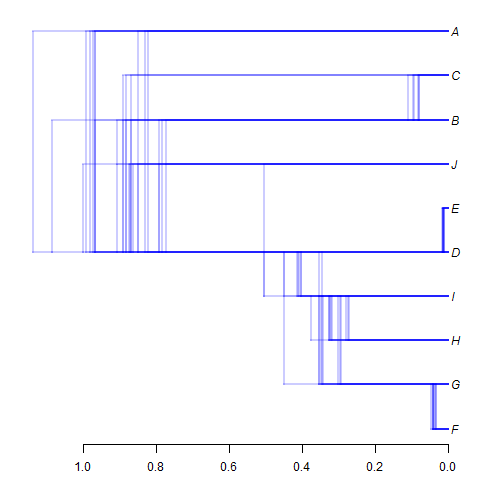Solution 2: same, but fix to constant depth.

``````rescale.tree<-function(tree,scale){
tree\$edge.length<-tree\$edge.length/max(nodeHeights(tree))*scale
tree
}
rescaled<-lapply(trees,rescale.tree,scale=1)
plotTree(rescaled[],direction="leftwards")
``````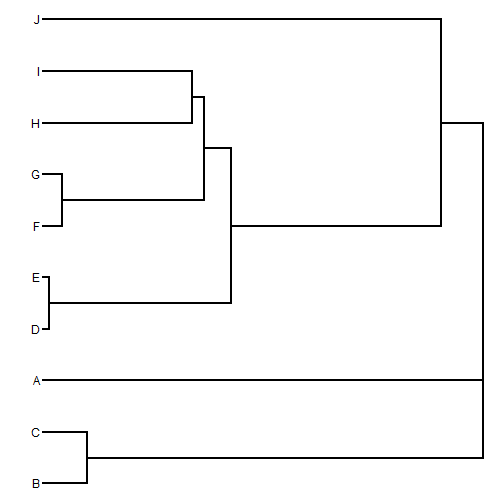``````xlim<-get("last_plot.phylo",envir=.PlotPhyloEnv)\$x.lim
## set tip order based on a majority rule consensus tree
tips<-setNames(1:Ntip(rescaled[]),
## plot our trees
for(i in 1:length(rescaled)){
plotTree(rescaled[[i]],color=make.transparent("blue",0.2),
mar=c(4.1,1.1,1.1,1.1),nodes="inner",
ftype=if(i==1) "i" else "off")
if(i==1) axis(1)
}
``````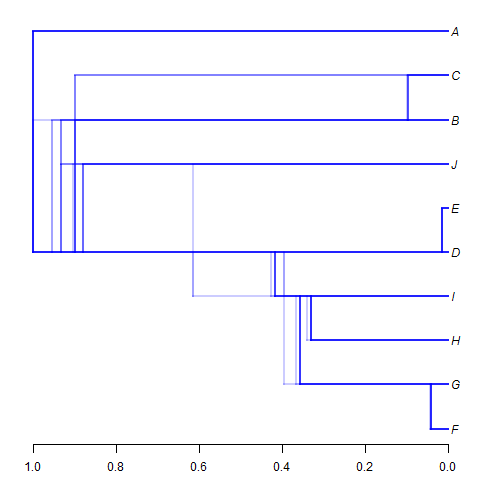Solution 3: same as #1, but using different colors.

``````## get depths of the tree
h<-sapply(trees,function(x) max(nodeHeights(x)))
## test plot the longest tree to get the maximum x dimension
plotTree(trees[[which(h==max(h))]],direction="leftwards")
````````````xlim<-get("last_plot.phylo",envir=.PlotPhyloEnv)\$x.lim
## set tip order based on a majority rule consensus tree
tips<-setNames(1:Ntip(trees[]),
## set colors
colors<-rainbow(n=length(trees))
## plot our trees
for(i in 1:length(trees)){
plotTree(trees[[i]],color=make.transparent(colors[i],0.4),
mar=c(4.1,1.1,1.1,1.1),nodes="inner",
ftype=if(i==1) "i" else "off")
if(i==1) axis(1)
}
``````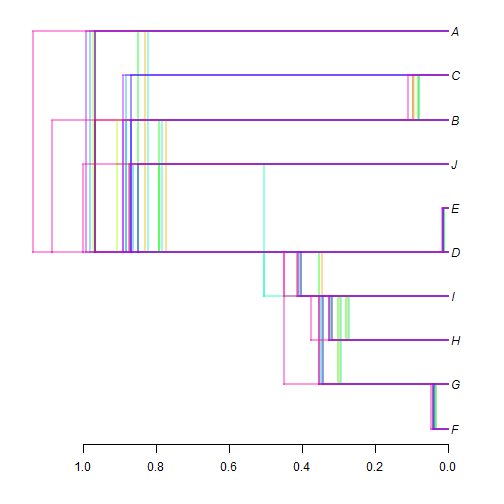Solution 4: same as #3, but with a slight vertical offset of each tree.

``````ylim<-c(0,Ntip(trees[])+1)
## get depths of the tree
h<-sapply(trees,function(x) max(nodeHeights(x)))
## test plot the longest tree to get the maximum x dimension
plotTree(trees[[which(h==max(h))]],direction="leftwards")
````````````xlim<-get("last_plot.phylo",envir=.PlotPhyloEnv)\$x.lim
## set tip order based on a majority rule consensus tree
tips<-setNames(1:Ntip(trees[]),
## set colors
colors<-rainbow(n=length(trees))
## plot our trees
for(i in 1:length(trees)){
y.shift<-(i-median(1:length(trees)))/length(trees)/2
plotTree(trees[[i]],color=make.transparent(colors[i],0.4),
mar=c(4.1,1.1,1.1,1.1),nodes="inner",
ftype=if(i==floor(median(1:length(trees)))) "i" else "off",
ylim=ylim)
if(i==1) axis(1)
}
``````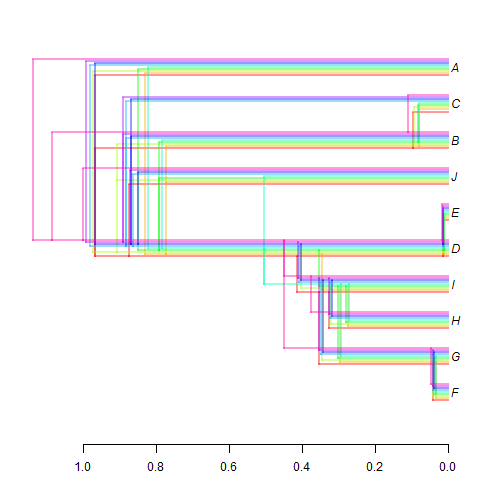Solution 5: same as #4, but with a fixed total depth.

``````rescale.tree<-function(tree,scale){
tree\$edge.length<-tree\$edge.length/max(nodeHeights(tree))*scale
tree
}
rescaled<-lapply(trees,rescale.tree,scale=1)
ylim<-c(0,Ntip(rescaled[])+1)
## get depths of the tree
h<-sapply(rescaled,function(x) max(nodeHeights(x)))
## test plot the longest tree to get the maximum x dimension
plotTree(rescaled[[i]],direction="leftwards")
````````````xlim<-get("last_plot.phylo",envir=.PlotPhyloEnv)\$x.lim
## set tip order based on a majority rule consensus tree
tips<-setNames(1:Ntip(rescaled[]),
## set colors
colors<-rainbow(n=length(rescaled))
## plot our trees
for(i in 1:length(rescaled)){
y.shift<-(i-median(1:length(rescaled)))/length(rescaled)/2
plotTree(rescaled[[i]],color=make.transparent(colors[i],0.4),
mar=c(4.1,1.1,1.1,1.1),nodes="inner",
ftype=if(i==floor(median(1:length(rescaled)))) "i" else "off",
ylim=ylim)
if(i==1) axis(1)
}
``````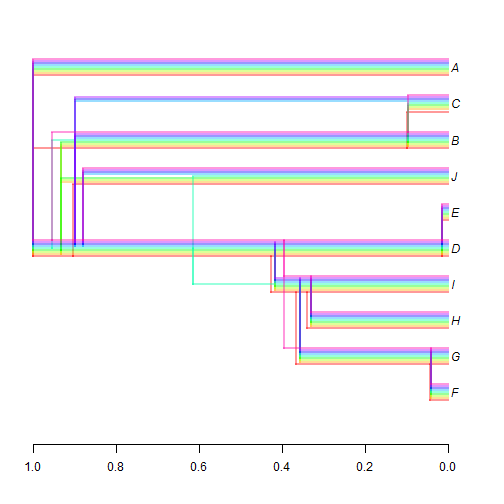We don't need to use “inner” node positions, I just prefer that because I feel that it makes it easier to visualize similarity of topology. Here is one final solution (#6) using “standard” node positions:

``````rescale.tree<-function(tree,scale){
tree\$edge.length<-tree\$edge.length/max(nodeHeights(tree))*scale
tree
}
rescaled<-lapply(trees,rescale.tree,scale=1)
ylim<-c(0,Ntip(trees[])+1)
## get depths of the tree
h<-sapply(rescaled,function(x) max(nodeHeights(x)))
## test plot the longest tree to get the maximum x dimension
plotTree(rescaled[[i]],direction="leftwards")
````````````xlim<-get("last_plot.phylo",envir=.PlotPhyloEnv)\$x.lim
## set tip order based on a majority rule consensus tree
tips<-setNames(1:Ntip(rescaled[]),
## set colors
colors<-rainbow(n=length(rescaled))
## plot our trees
for(i in 1:length(rescaled)){
y.shift<-(i-median(1:length(rescaled)))/length(rescaled)/2
plotTree(rescaled[[i]],color=make.transparent(colors[i],0.4),
mar=c(4.1,1.1,1.1,1.1),
ftype=if(i==floor(median(1:length(rescaled)))) "i" else "off",
ylim=ylim)
if(i==1) axis(1)
}
``````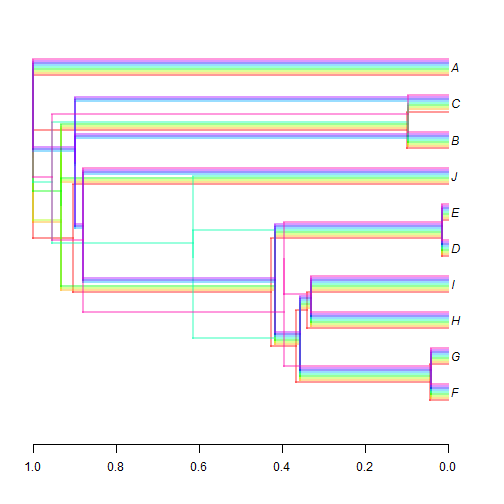That's it.

BTW, the trees used in this exercise were simulated to be similar but different using the following code with phangorn:

``````library(phangorn)
true.tree<-pbtree(n=10,tip.label=LETTERS[1:10])
D<-cophenetic(true.tree)
trees<-lapply(trees,nnls.tree,dm=D,rooted=TRUE)
class(trees)<-"multiPhylo"
rescale.tree<-function(tree,scale){
tree\$edge.length<-tree\$edge.length/max(nodeHeights(tree))*scale
tree
}
h<-rnorm(n=length(trees),mean=1,sd=0.1)
trees<-mapply(rescale.tree,tree=trees,scale=h,SIMPLIFY=FALSE)
class(trees)<-"multiPhylo"
``````

[Ed. - A previous version of this post had `ylim=c(0,11)` rather than `ylim<-c(0,Ntip(trees[])+1)` & `ylim=ylim` as in the current edit. Sorry about that!]

[Ed. #2 - I have also changed `i==median(1:length(trees))` to `i==floor(median(1:length(trees)))` to address cases of an even number of trees in which the medianth tree is not an integer!]

#### 1 comment:

1.Note: due to the very large amount of spam, all comments are now automatically submitted for moderation.